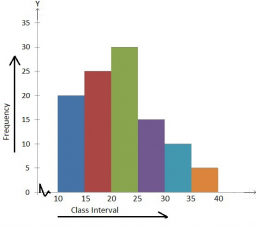# A sample

A sample of 10 randomly selected students revealed the following grades in
Business Statistics first test (in a marking scale of 0 to 100): 79, 63, 60, 45, 55, 58, 59, 62, 40, 68. Examine the data using groups and presenting:
a) the Histogram of the absolute frequencies and the polygon;
b) the Histogram of the relative frequencies;
c) the Histogram of the cumulative frequencies and the ogive;
d) the Mean;
e) the Median;
f) the Mode;
g) the Variance;
h) the Standard deviation;
i) the Coefficient of Variance;
j) the Coefficient of Pearson and discuss the skewness briefly.
Make a table consisting of columns with the necessary calculations concerning the above
information.

m =  58.9

### Step-by-step explanation:Did you find an error or inaccuracy? Feel free to write us. Thank you!

Tips for related online calculators
Looking for help with calculating arithmetic mean?
Looking for a statistical calculator?
Looking for a standard deviation calculator?

#### Grade of the word problem:

We encourage you to watch this tutorial video on this math problem: